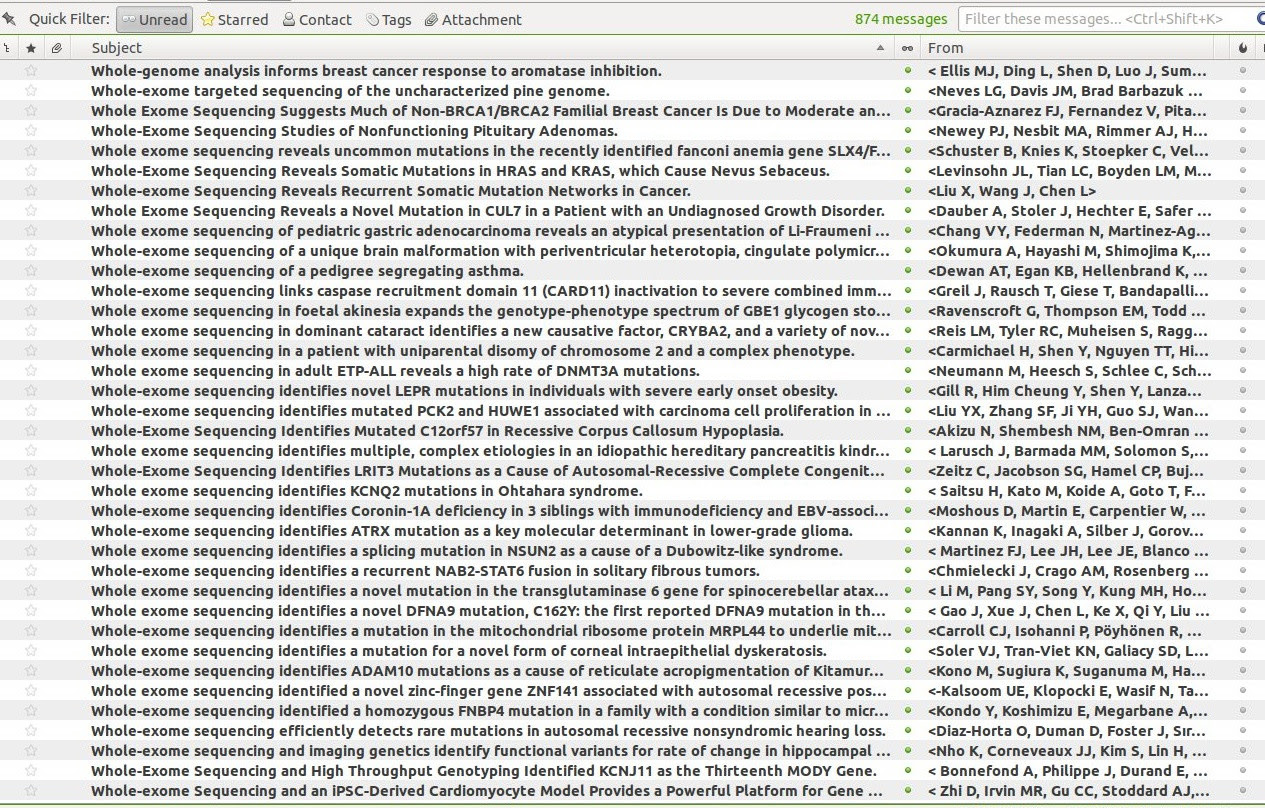## 15 June 2013

### Dear editors,...

Here is a screenshot of Thunderbird with the RSS feeds for "NCBI/pubmed: Exome Sequencing".I'm pretty sure that all that (semantic) information is lost.

Dear editors, could you please ask the authors to complete or create an article in wikipedia about their paper once you have published it (possibly using a semantic template ?).

Thank you,

Pierre

See also We found a gene involved in a genetic disease. Now, what is the TODO list ?

## 09 June 2013

### How to fit a sentence in a rectangle with the Hershey vectorial font.

via wikipedia: The Hershey fonts are a collection of vector fonts developed circa 1967 by Dr. A. V. Hershey (...). Vector fonts are easily scaled and rotated in two or three dimensions; consequently the Hershey fonts have been widely used in computer graphics and computer-aided design programs.. When programming, I often have to fit a sentence in a rectangle (for example to write the name of a short-read in the graphical view of a BAM) so I wrote a XML version of the hershey font.

```<?xml version="1.0"?>
<hershey>
<letter id="1" count="9" left="-5" right="5" char="a">
<moveto x="0" y="-5"/>
<lineto x="-4" y="4"/>
<moveto x="0" y="-5"/>
<lineto x="4" y="4"/>
<moveto x="-2" y="1"/>
<lineto x="2" y="1"/>
</letter>
<letter id="2" count="16" left="-5" right="5" char="b">
<moveto x="-3" y="-5"/>
<lineto x="-3" y="4"/>
<moveto x="-3" y="-5"/>
<lineto x="1" y="-5"/>
<lineto x="3" y="-4"/>
<lineto x="3" y="-2"/>
<lineto x="1" y="-1"/>
<moveto x="-3" y="-1"/>
<lineto x="1" y="-1"/>
<lineto x="3" y="0"/>
<lineto x="3" y="3"/>```
From there, I can generate some bindings
for various programming languages using XSLT, for example javascript:
```{
"1":[{t:'M',x:0,y:-5},{t:'L',x:-4,y:4},{t:'M',x:0,y:-5},{t:'L',x:4,y:4},{t:'M',x:-2,y:1},{t:'L',x:2,y:1}],
"2":[{t:'M',x:-3,y:-5},{t:'L',x:-3,y:4},{t:'M',x:-3,y:-5},{t:'L',x:1,y:-5},{t:'L',x:3,y:-4},{t:'L',x:3,y:-2},{t:'L',x:1,y:-1},{t:'M',x:-3,y:-1},{t:'L',x:1,y:-1},{t:'L',x:3,y:0},{t:'L',x:3,y:3},{t:'L',x:1,y:4},{t:'L',x:-3,y:4}], ...
```

In the Javascript example below, I'm generating some random rectangles where a sentence is written:

That's it,
Pierre